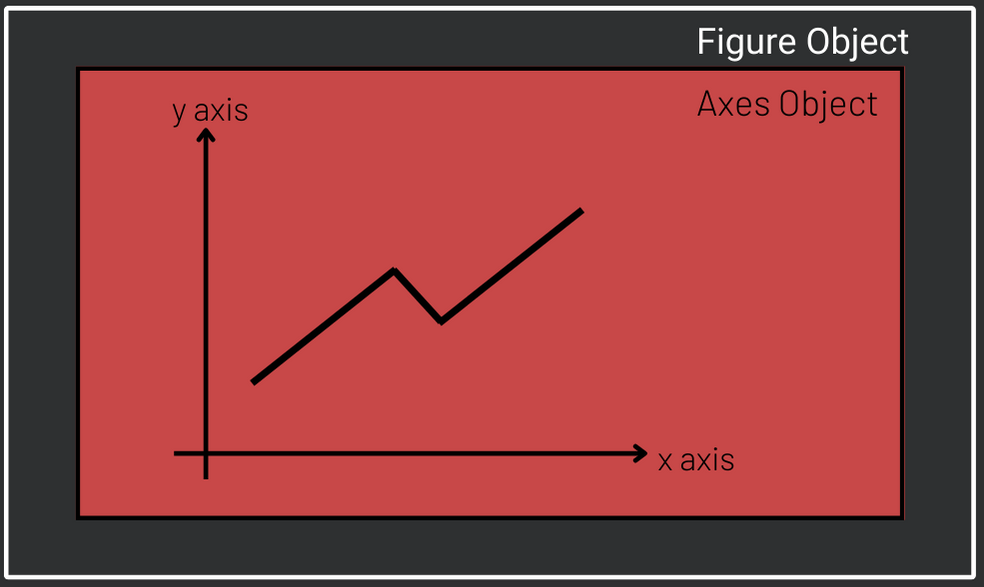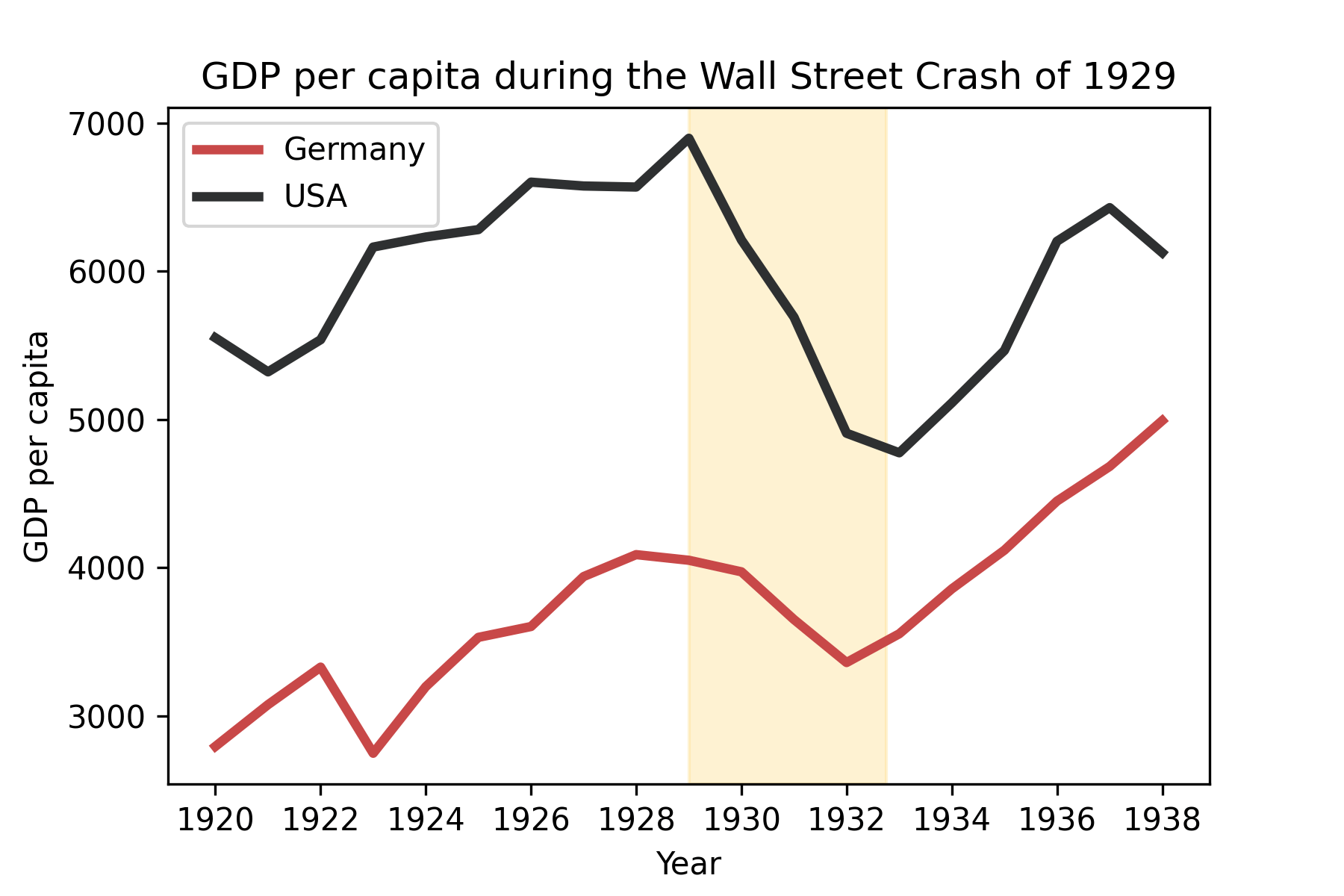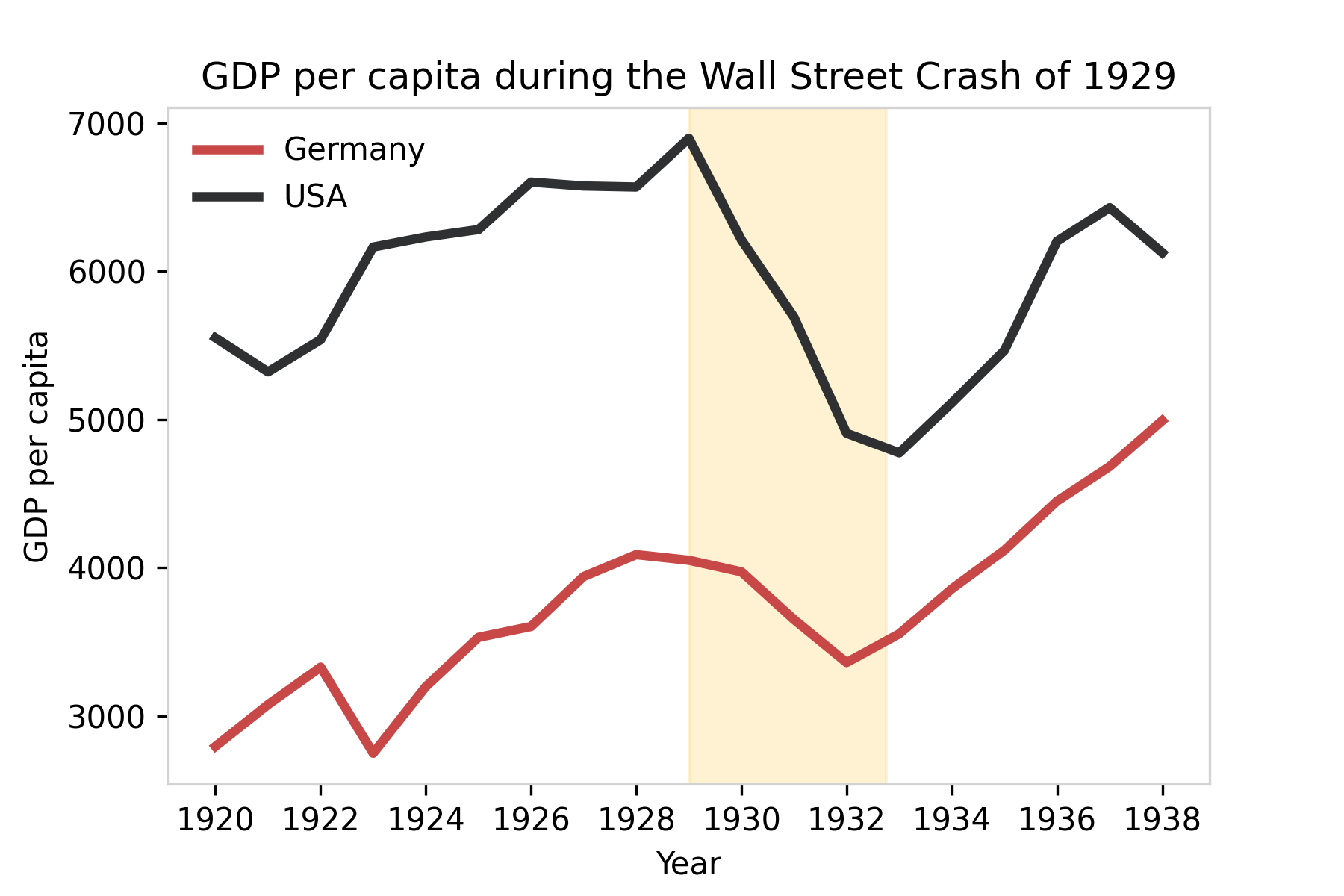# Introduction

‘All that is solid melts into the air’

Karl Marx and Friedrich Engels

As a historian and social scientist I often find it challenging to customize my Python plots and make them clear and compelling. What is a Python class, object or method? 😲 In this lesson, you will learn what those concepts are and how to use them to create effective graphs with Matplotlib. We will plot and highlight elements in the trend of Gross Domestic Product (GDP) per capita of Germany and the USA during the 1929 crisis. If you would like to know more about this event, check this out.

# Data source

Data used in this lesson is available at Harvard Business School

# Coding the past: beautiful visualizations with Matplotlib

## 1. What is Matplotlib?

Matplotlib is a Python library aimed at creating visualizations. It has a good interface with pandas dataframes, which makes it very practical to use. Matplotlib is the base library for other visualization libraries, like Seaborn.

Before we dive into Matplotlib, we have to understand the concept of class and object. A Python object could be a plot, a string of text or a dataframe, for example. ‘Classes are a sort of blueprint for objects, specifying their properties (attributes) and behaviors (methods). For example, an object of the Matplotlib class Axes will store a plot and will always have an x axis label attribute. The class Axes might give origin to several different objects (plots), all with an x axis label property, but the content of this property is particular to each plot. We say we instantiate an object of a certain class when we create it.

There are many ways you can use Matplotlib, but in order to be able to customize your plot, it is recommended to use the Matplotlib subplots() method. It creates two objects: one object of the class Figure, usually called fig and one object of the class Axes, usually called ax. The former is a sort of container where your plot will be created. The latter is the plot itself. Note that the Axes object is contained in the Figure object. Refer to the Matplotlib documentation for further details.Download the data file here. To read the data, use the pandas method pd.read_csv(), which takes 3 parameters. The first is the file path. The second is index_col and it tells pandas which column should be the index of the data frame. Finally, parse_dates set to True converts the index into date format. In the code below, data is loaded and one dataframe is created for each country with the pandas method loc.

content_copy
Copy

## 2. Matplotlib basic plot

Although in this lesson our fig object will have only one plot, it might have more. Most of the customization will be made through ax methods. To start we will call the ax method plot() twice to create our plots. Note that plot()’s first argument contains the dates and is plotted on the x axis while the second, containing the GDP, is plotted on the y axis. Finally, we show the plot.

content_copy
Copy## 3. Restricting time span

One important aspect to consider when you tell a story with a plot is what you would like to highlight. In this plot, we want to highlight the effect of the 1929 crisis on GDP per capita rather than the effect of the Second or First World War. Thus, let us restrict our time span to the period 1920/1938. Note that when your index is a date you can use pandas loc to specify a certain period of the data:

content_copy
Copy## 4. Adding a Matplotlib legend

To add a legend, first you have to label each of the line plots and then call the legend() method of ax. Quite intuitive, right?

content_copy
Copy## 5. Adding a title and and labels to matplotlib axes

There are three methods of ax to set title and labels. They start with set followed by the title or label they set: set_xlabel, set_ylabel, set_title.

content_copy
Copy## 6. Changing line colors

One way of creating your own color palette is with a Python list containing the colors you would like to use. This page has smart recommendations on the use of colors. In this case, a diverging color was chosen to distinguish between the two countries. Color is an argument of plot() and colors are selected by the list index.

content_copy
Copy## 7. Highligthing areas and elements with axvspan

In this step, we start by increasing the line width of both trends to 3. After that, we would like to highlight the period of crisis. For that we use the method axvspan(xmin, xmax, ymin=0, ymax=1, ...) in which we specify the start and end date of the desired period. The y axis is not specified because, by default, the highlighted area goes from zero to the maximum value of y. alpha adds a degree of transparency to the region highlighted.

content_copy
Copy## 8. Eliminating the frame of matplotlib legend

Edward Tufte, an expert in the field of data visualization, introduced the concept of data-ink ratio in the book The Visual Display of Quantitative Information. Data-ink ratio is the proportion of ink in a plot used to display non-redundant data. The author recommends maximizing this ratio as much as possible to make your plot clearer and to avoid distracting your reader.

$data \: ink \: ratio = {data \: ink \over total \:ink}$

In order to improve our data-ink ratio, we will eliminate the legend frame. This can be done by setting framon parameter to false inside the legend() method.

The frame around the plot is made by objects of the class Spine. Print ax.spines and note that you have 4 spines (left, right, bottom, top). It would be nice to have this frame in a lighter color so that it does not call so much attention. This can be done by the set_edgecolor() method . To set all of them to the same color, we can iterate them in ax.spines.values() and set one by one to light gray:

content_copy
CopyIn the next lessons you will learn how to further improve graphs like this. Thanks for reading! 😊

# Conclusions

• To customize your plot, use Matplotlib method subplots();
• Subplots() creates two objects: one of the class Figure, usually called fig and one of the class Axes, usually called ax;
• Use Axes methods to shape your plot according to your needs.